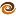# EmptyCrate

#### About Me | Contact Me | Training |ChaiScript.com | YouTube Channel

Pass by iterator is not a new concept, but one that we are going to perhaps give a new name to today and propose as a standard for normal usage. If you take for example the normal way of passing around standard containers:

``````// Print a vector of strings separated by spaces.
void print(const std::vector &t_vector)
{
for(std::vector::const_iterator itr = t_vector.begin();
itr != t_vector.end();
++itr)
{
std::cout << *itr << " ";
}
}

std::vector m_vec;
m_vec.push_back("Hello");
m_vec.push_back("World");
print(m_vec);
``````

The idea of “Pass By Iterator” that we are proposing involves never again writing code like the above. With this example if you wanted to change your container to an `std::list` or an `std::set` you would have to modify several lines of code in the `print()` function. If, however, you had passed a range of iterators to print in the first place your code would be reusable, more succinct and, most importantly, more open to refactoring.

``````//Print a range of iterators separated by spaces.
template
void print(InItr begin, InItr end)
{
while (begin != end)
{
std::cout << *begin << " ";
++begin;
}
}

std::vector m_vec;
m_vec.push_back("Hello");
m_vec.push_back("World");
print(m_vec.begin(), m_vec.end());
``````

This technique can be used everywhere you pass containers around in your code. One downside is that it will probably be more difficult to debug your compilation errors. The concept of passing around containers as a pair of iterators is how the standard library was designed. One way to think about it is that you are adding to your own personal library of algorithms.

My Videos

Related